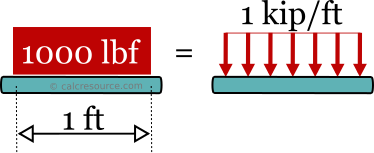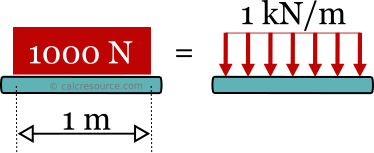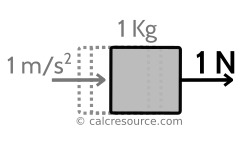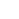## kN/m - kips/ft converter

Enter the distributed load in either of the next two fields and get it converted instantly, from kilonewton per meter to kilopounds per foot (kN/m to kips/ft), or reversely, from kilopounds per foot to kilonewton per meter (kips/ft to kN/m).

### Conversion Formulas

1 kip/ft ≈ 14.5939 kN/m

1 kN/m ≈ 0.068521 kips/ft

#### Precise conversion:

1 kip/ft = 14.5939029372063648294 kN/m

#### Rule of thumb:

1 KN/m ≈ 1/15 kip/ft

## Definitions

### General

Distributed forces, represent a widely occurring pattern in structural mechanics. They may be distributed over a length, which is typical for frame structures, where the members (beam and columns) are idealized as linear elements, or over a surface area, which is common for floors and walls. In the first case, distributed forces are called linear loads, while on the second case, surface loads. This page is about linear loads, and in particular, about two of the most widely used units for measuring linear loads, the kN/m from the metric system (SI), and the kips/ft from the imperial system.

Linear loads can be uniform or varying. A uniform distributed load (abbr. UDL) has a constant magnitude over the length it is distributed. On the other hand, a varying distributed load (abbr. VDL) features a varying magnitude. Regardless, linear loads, uniform or varying, are all measured with the same units.

### The kilopound per foot [kips/ft]

The kilopound per foot (symbolized kips/ft or kip/ft) is a unit of a force, that is distributed over a length. It is defined as kilopound [kip], which is one thousand pounds [lbf] (a unit of force), divided by foot [ft], which is a unit of length. In other words, the kip/ft is equivalent to a 1000 pound force [lfb] distributed over 1 foot distance.A distributed load of 1 kips/ft is equivalent to a 1000 pound force, distributed over 1ft length

The definition of pound force [lbf] depends on the force of gravity on the earth's surface upon a mass equal to one avoirdupois pound. Converted to the respective metric unit, the Newton [N] it becomes: 1 lbf = 4.4482216152605 N. The above definition depends on the earth's gravitational acceleration g, which varies from place to place, but is conventionally averaged to g ≈ 32.1740 ft/s2, at sea level.A force of 1 lbf corresponds to the weight of one pound mass at sea level

Foot [ft] is a unit of length for the Imperial and US customary system of units. It was historically conceived as the length of a human male foot. Today, however, it is conventionally defined, using the metre SI unit, like this:

1ft = 0.3048m

The conversion rule between kilopound per foot and kilonewton per meter (kip/ft to kN/m) can be found, using the conversion rules between the two components of the unit: the force and the length. It is:

1 lbf = 4.4482216152605 N

Therefore, 1 kip, which is 1000 pound becomes also:

1 kip = 4.4482216152605 KN

As a result, 1kip / 1ft would be:

1kip / 1ft = 4.4482216152605KN / 0.3048m

Finally:

1kip/ft = 14.5939029372063648294 kN/m

This is the conventionally equivalent of 1 kip/ft in kN/m.

#### Kilopound per foot and other units of distributed force

The relationship between kilopound per foot [kip/ft] and other units of linearly distributed force, are shown in the following table (i.e. how many kip/ft makes every unit in the table). The values in the table are not exact (except for those in italics):

If you wonder how to use the above table, here is a quick example. Assuming, you need to find the relationship between Newton per centimetre [N/cm] and kilopound per foot [kip/ft], the following equation is obtained from the table:

1 N/cm = 6.85218 10-3 kips/ft

or

1 N/cm = 0.00685218 kips/ft

### The kilonewton per meter [kN/m]

The kilonewton per meter (symbolized kN/m or KN/m) is a unit of linearly distributed force, compatible with the SI (Systeme International), system of units. It is defined as a kilonewton [kN], which is one thousand Newtons [N], divided by meter [m]. The kN/m is equivalent to a force of 1000 Newton distributed over 1 meter length.A distributed load of 1 kN/m is equivalent to a 1000 Newton force, distributed over 1m length

Newton [N] is a unit of force according to the SI system of units. It is a derived unit, based on the kilogram [Kg], a unit of mass, the metre [m], a unit of length and the second [s], a unit of time. It is defined as the force required to accelerate a mass equal to 1 Kg by 1 m/s2, according to the Newton's second law of motion:

where the force, the mass and the acceleration.A force of 1 Newton accelerates with 1m/s2 a mass of 1kg

Using the Newton's second law of motion with earth's gravitational acceleration g (for this purpose approximated to g ≈ 9.806 m/s2), we can relate the Newton force unit with the weight of a unit mass (equal to 1 kg). We obtain:

1 kg x 9.807 m/s2 ≈ 9.807 N

or

1 N ≈ 0.102 kg m/s2

This means, that a force of 1 N, is equivalent to the weight of a mass equal to 102 gr, at earth's sea level. Similarly, 10 N are equivalent to the weight of 1.02 kg mass. These are rules of thumb, useful for many practical purposes. In reality however, earth's gravity depends on the location (altitude and geographical latitude), and cannot be considered an invariant constant.

Meter or metre [m] is the base unit of length for the SI system of units. It was originally conceived as 1/10,000,000 of the distance along earth's meridian from the equator to the North Pole (a full longitude circle of earth is approximately equal to 40,000 km). Today, it is defined as the distance traveled by light in vacuum in seconds. This definition offers the advantage of invariance through space and time but on the other hand is impractical for everyday use.

#### Kilonewton per metre and other units of distributed force

The relationship between kilonewton per meter [kN/m] and other units of linearly distributed force is presented in the following table (i.e. how many kN/m makes each one of the units in the table). The values in the table are not exact (except for those in italics):

To demonstrate how to use the above table, a quick example is given here. Assuming, you need to find the relationship between pound force per inch [lbf/in] and kilonewton per meters [kN/m], the following equation is obtained from the table:

1 lbf/in = 0.175127 KN/m

### How to convert from kilopounds per foot to kilonewton per meter (kip/ft to kN/m)

• Multiply distributed load in kips/ft with 14.5939
• The result is the load in kN/m

For example to convert 2.5 kip/ft to kN/m we have: 2.5x14.5939 ≈ 36.48 kN/mFor a precise conversion multiply kips/ft with 14.5939029372063648294 to obtain kN/m

### How to convert from kilonewton per meter to kilopounds per foot (kN/m to kip/ft)

• Divide distributed load in kN/m by 14.5939
• The result is the load in kip/ft

For example to convert 50 kN/m to kips/ft, we should do: 50/14.5939 ≈ 3.426 kips/ft

### Conversion table from kilonewton per meter to kilopounds per foot (KN/m to kips/ft)

In the following table some typical load values in kN/m are converted to kips/ft. These conversions are approximated with 4 significant digits. More precise values can be obtained from the calculator in the top of the page.

### Conversion table from kilopounds per foot to kilonewton per meters (kips/ft to kN/m)

In the following table some typical load values in kips/ft are converted to kN/m. These conversions are approximated with 4 significant digits. More precise values can be obtained from the calculator in the top of the page.